# 虹膜弹性运动的不对等性Inhomogeneity of Iris Elastic Motion

• 全文下载: PDF(840KB)    PP.721-729   DOI: 10.12677/CSA.2018.85080
• 下载量: 521  浏览量: 772

With the development of image acquisition equipment, the accuracy of iris recognition for feature point positioning is higher and higher, and the traditional rubber plate model cannot meet the requirements of iris recognition on accuracy. In order to weaken the effect of pupil dilatation and contraction on the recognition results, an iris external and inward elasticity model is proposed according to the different structure of the open major muscle and the sphincter. This model starts with the tissue dynamics and analyzes the importance of the stress and shear stress in the deformation process by combining the characteristics of the elastic changes of iris tissue under different moving states. The inhomogeneity of the expansion and contraction of iris tissue is studied in depth.

1. 引言

2. 本文模型

2.1. 房水折射Figure 1. Iris tissue structure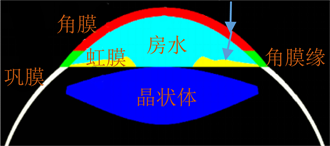Figure 2. Aqueous humor refraction section diagram

2.2. 虹膜动力学定位

$\begin{array}{l}{\epsilon }_{r}=\frac{\partial {u}_{r}}{\partial r}-\frac{1}{2}\left\{{\left(\frac{\partial {u}_{r}}{\partial r}\right)}^{2}+{\left(\frac{\partial {u}_{\theta }}{\partial r}\right)}^{2}\right\}\\ {\epsilon }_{\theta }=\frac{1}{r}\frac{\partial {u}_{\theta }}{\partial \theta }+\frac{{u}_{r}}{r}-\frac{1}{2{r}^{2}}\left\{{\left(\frac{\partial {u}_{\theta }}{\partial \theta }\right)}^{2}+{\left(\frac{\partial {u}_{r}}{\partial \theta }\right)}^{2}-2\frac{\partial {u}_{r}}{\partial \theta }{u}_{\theta }+2\frac{\partial {u}_{\theta }}{\partial \theta }{u}_{r}+{u}_{r}^{2}+{u}_{\theta }^{2}\right\}\\ {\gamma }_{r\theta }=\frac{1}{r}\frac{\partial {u}_{r}}{\partial \theta }+\frac{\partial {u}_{\theta }}{\partial r}-\frac{{u}_{\theta }}{r}-\frac{1}{r}\left\{\left(\frac{\partial {u}_{r}}{\partial \theta }\right)\left(\frac{\partial {u}_{r}}{\partial r}\right)+\left(\frac{\partial {u}_{\theta }}{\partial r}\right)\left(\frac{\partial {u}_{\theta }}{\partial \theta }\right)+\frac{\partial {u}_{\theta }}{\partial r}{u}_{r}-\frac{\partial {u}_{r}}{\partial r}{u}_{\theta }\right\}\end{array}$ ,(1)

$\left(\begin{array}{c}{\epsilon }_{r}\\ {\epsilon }_{\theta }\\ {\gamma }_{r\theta }\end{array}\right)=\left(\begin{array}{ccc}\frac{1}{{E}_{r}}& -\frac{{\nu }_{r\theta }}{{E}_{\theta }}& 0\\ -\frac{{\nu }_{\theta r}}{{E}_{r}}& \frac{1}{{E}_{\theta }}& 0\\ 0& 0& \frac{2\left(1+{\nu }_{r\theta }\right)}{{E}_{\theta }}\end{array}\right)\left(\begin{array}{c}{\sigma }_{r}\\ {\sigma }_{\theta }\\ {\tau }_{r\theta }\end{array}\right)$ , (2)

$\frac{{\nu }_{\theta r}}{{E}_{r}}=\frac{{\nu }_{r\theta }}{{E}_{\theta }}$ ,(3)

${f}_{CD}\left(r+\text{d}r\right)\text{d}\theta -{f}_{AB}r\text{d}\theta +{f}_{AD}\text{d}r\mathrm{sin}\left(-\frac{\text{d}\theta }{2}\right)-{f}_{BC}\text{d}r\mathrm{sin}\frac{\text{d}\theta }{2}+{g}_{AD}\text{d}r\mathrm{cos}\frac{\text{d}\theta }{2}-{g}_{BC}\text{d}r\mathrm{cos}\frac{\text{d}\theta }{2}=0$ , (4)

2.3. 虹膜扩张和收缩运动的不对等性

${f}_{CD}\left({\sigma }_{r}\right)=|{\sigma }_{r}|+\frac{\partial {\sigma }_{r}}{\partial r}\text{d}r$

${g}_{CD}\left({\sigma }_{r}\right)=|{\sigma }_{r}|+\frac{\partial {\sigma }_{r}}{\partial r}\text{d}r$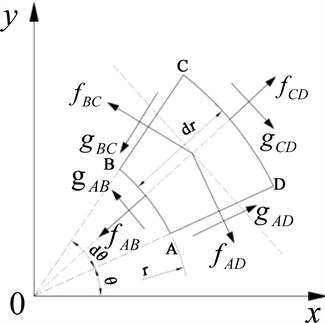Figure 3. Iris characteristic point force analysis diagram

$\begin{array}{l}{f}_{BC}=|{\sigma }_{\theta }|+\frac{\partial {\sigma }_{\theta }}{\partial \theta }\text{d}\theta \\ {g}_{BC}=|{\tau }_{\theta r}|+\frac{\partial {\tau }_{\theta r}}{\partial \theta }\text{d}\theta \end{array}$

$\frac{|{\sigma }_{r}|-|{\sigma }_{\theta }|}{r}+\frac{\partial {\sigma }_{r}}{\partial r}+\frac{\text{1}}{r}\frac{\partial {\tau }_{r\theta }}{\partial r}=0$ , (5)

$\frac{{\sigma }_{r}-{\sigma }_{\theta }}{r}+\frac{\partial {\sigma }_{r}}{\partial r}+\frac{\text{1}}{r}\frac{\partial {\tau }_{r\theta }}{\partial r}=0$ , (6)

(1)(2)代入上式，化简可得如下控制微分方程边值问题：

$\left\{\begin{array}{l}\frac{{\text{d}}^{2}u}{\text{d}{r}^{2}}+\frac{1}{r}\frac{\text{d}u}{\text{d}r}-\frac{\xi u}{{r}^{2}}-\frac{1-\nu \xi }{2r}{\left(\frac{\text{d}u}{\text{d}r}\right)}^{2}-\frac{\nu -1}{2r}{\left(\frac{u}{r}\right)}^{2}-\frac{1}{2}\frac{\text{d}}{\text{d}r}{\left(\frac{\text{d}u}{\text{d}r}\right)}^{2}-\frac{\nu \xi }{2}\frac{\text{d}}{\text{d}r}{\left(\frac{u}{r}\right)}^{2}=0\\ u\left({r}_{1}\right)={\mu }_{1}\ge 0,\text{\hspace{0.17em}}\text{\hspace{0.17em}}u\left({r}_{2}\right)=0,\text{\hspace{0.17em}}\text{\hspace{0.17em}}r\in \left({r}_{1},{r}_{2}\right)\end{array}$ ,(7)

$\frac{-{\sigma }_{r}+{\sigma }_{\theta }}{r}+\frac{\partial {\sigma }_{r}}{\partial r}+\frac{\text{1}}{r}\frac{\partial {\tau }_{r\theta }}{\partial r}=0$ , (8)

$\left\{\begin{array}{l}\frac{{\text{d}}^{2}u}{\text{d}{r}^{2}}-\frac{2\xi -1}{r}\frac{\text{d}u}{\text{d}r}-\frac{\xi \left(1-2\nu \right)}{{r}^{2}}u-\frac{1-\nu \xi }{2r}{\left(\frac{\text{d}u}{\text{d}r}\right)}^{2}+\frac{\xi \left(3\nu -1\right)}{2r}{\left(\frac{u}{r}\right)}^{2}-\frac{1}{2}\frac{\text{d}}{\text{d}r}{\left(\frac{\text{d}u}{\text{d}r}\right)}^{2}-\frac{\nu \xi }{2{r}^{2}}\frac{\text{d}}{\text{d}r}{\left(u\right)}^{2}=0\\ u\left({r}_{1}\right)={\mu }_{1}<0,\text{\hspace{0.17em}}\text{\hspace{0.17em}}u\left({r}_{2}\right)=0,\text{\hspace{0.17em}}\text{\hspace{0.17em}}r\in \left({r}_{1},{r}_{2}\right)\end{array}$ ,(9)

3. 实验结果与分析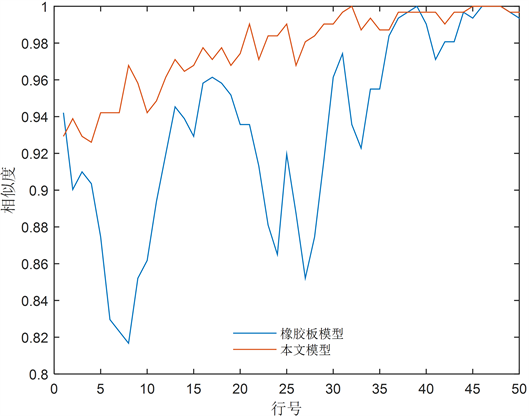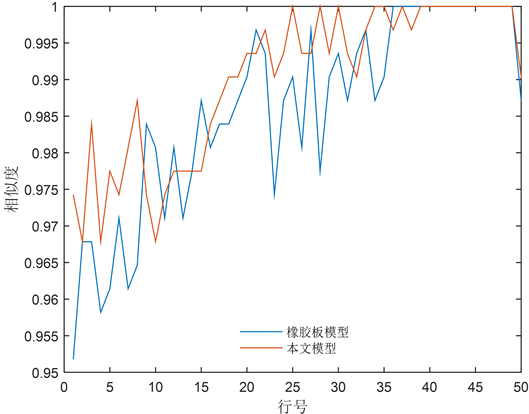Figure 4. Normalized one-line ratio of the same gray value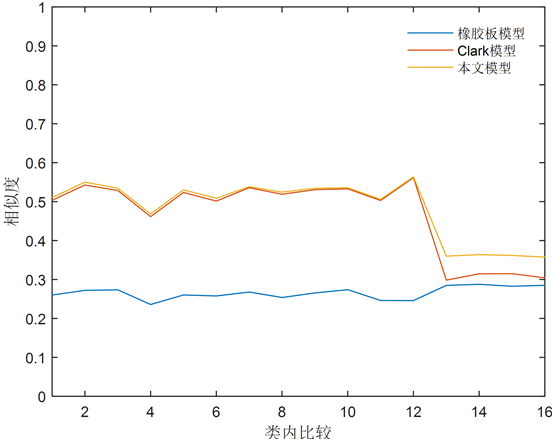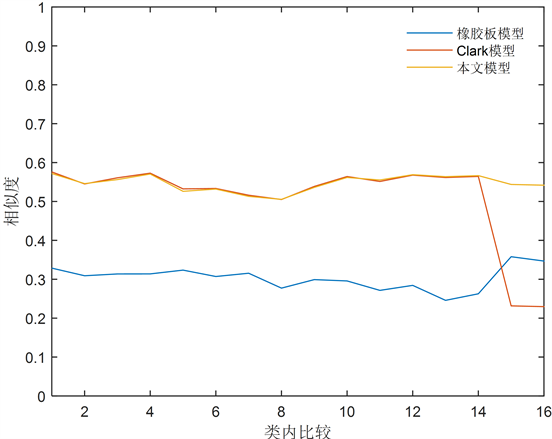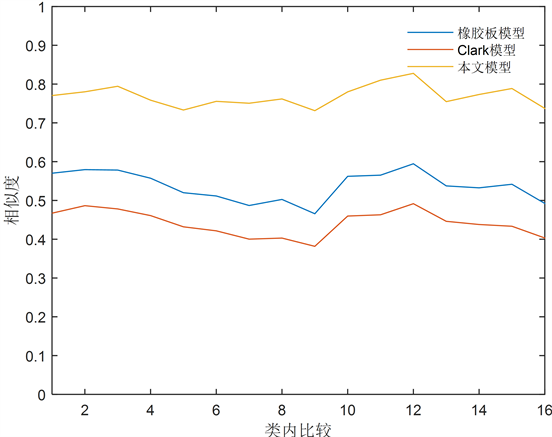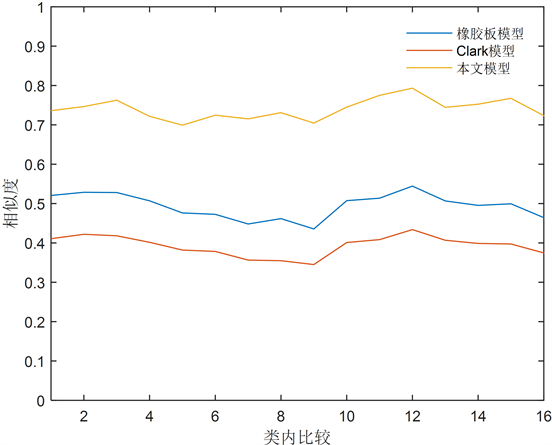Figure 5. Inner structure similarity of iris image

4. 结语

  Jain, A.K., Flynn, P. and Ross, A.A. (2008) Handbook of Biometrics. Springer, US. https://doi.org/10.1007/978-0-387-71041-9  Daugman, J. (2004) How Iris Recognition Works. International Conference on Image Processing, 1, I-33-I-36.  Wyatt, H.J. (2000) A “Minimum-Wear-and-Tear” Meshwork for the Iris. Vision Research, 40, 2167-2176. https://doi.org/10.1016/S0042-6989(00)00068-7  Yuan, X. and Shi, P. (2005) A Non-Linear Normalization Model for Iris Recognition. Advances in Biometric Person Authentication. Springer, Berlin, Heidelberg, 135-141.  Thornton, J., Savvides, M. and Kumar, B.V.K.V. (2007) A Bayesian Approach to Deformed Pattern Matching of Iris Images. IEEE Transactions on Pattern Analysis and Machine Intelligence, 29, 596-606. https://doi.org/10.1109/TPAMI.2007.1006  Clark, A.D., Kulp, S.A., Herron, I.H., et al. (2013) A Theoretical Model for Describing Iris Dynamics. Handbook of Iris Recognition. Springer, London, 129-150.  Santos-Villalobos, H.J., Barstow, D.R., Karakaya, M., et al. (2012) ORNL Biometric Eye Model for Iris Recognition. Fifth International Conference on Biometrics: Theory, Applications and Systems, Arlington, VA, 176-182.  Frigerio, E., Marcon, M., Sarti, A., et al. (2012) Correction Method for Nonideal Iris Recognition. IEEE International Conference on Image Processing, Orlando, FL, 30 September-3 October 2012, 1149-1152.  杨桂通. 弹性力学简明教程[M]. 北京: 清华大学出版社, 2013.  Dhir, L., Habib, N.E., Monro, D.M. and Rakshit, S. (2010) Effect of Cataract Surgery and Pupil Dilation on Iris Pattern Recognition for Personal Authentication. Eye, 224, 1006-1010. https://doi.org/10.1038/eye.2009.275  Ma, L., Tan, T., Wang, Y., et al. (2004) Efficient Iris Recognition by Characterizing Key Local Variations. IEEE Transactions on Image Processing, 13, 739-750. https://doi.org/10.1109/TIP.2004.827237  Montanino, A., Angelillo, M. and Pandolfi, A. (2017) Modelling with a Meshfree Approach the Cornea-Aqueous Humor Interaction during the Air Puff Test. Journal of the Mechanical Behavior of Biomedical Materi-als, 77, 205-216.  Mark, H.H. (2003) Aqueous Humor Dynamics and the Iris. Medical Hypotheses, 60, 305-308.